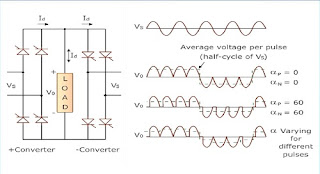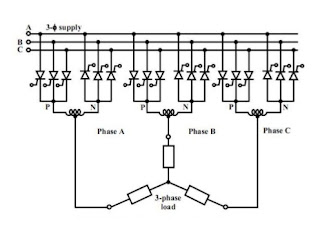## Page

### Cycloconverter operation

A principle of cyclo-converter operation :
• A cyclo-converter is a one types of device that converts AC power at a certain frequency into AC power but lower frequency.
• The principle of cyclo-converter is described given below by using a single phase to single phase step-up converters and single phase to single phase step down converters.Cyclo-converter operation
Types of cyclo-converter :
1. Single phase to single phase cyclo-converter
2. Three phases to three phase cyclo-converter

Single phase to single phase bridge type cyclo-converter
• The operation of bridge type cyclo-converter shown in the figure. It can be easily explained for both discontinuous and continuous load current. The voltage and current waveform would again be as shown in the figure.
• It consists of a total of eight thyristors, four for the positive group and four for the negative group.Bridge type single phase to single phase cyclo-converters
This converter having to back to back connection of full wave rectifier. We have to assume that get on forth of the input voltage as the output.  For the first two cycles of  VS the positive converters operate supplying current to the load and the input voltage get rectified. In the next two cycles, the other converter called the -ve converters, it will operate the current load in the opposite direction called a reverse direction. so we also conclude that one converter is an operation the other converter will be disabled conditions, therefore, there is no current circulating between rectifiers.

Three phases to three phase cyclo-converters

Out of the several configurations of 3 phase to 3 phase cyclo-configuration as shown in the figure. Here only one important scheme used for a large industrial drive is presented. Each phase of the 3 phase output must have a displacement of 120 degrees. The figure shows the circuit arrangement of 3-phase to three phase cyclo-converter using three sets of three-phase half wave circuit employing a total of 18 thyristors.3-phase to 3-phase cyclo-converters
Applications :

The application of cyclo-converters include the following :
• Speed control of high power ac drives.
• Static VAr compensation.
• Induction heating.
• Synchronous motor.
• Rolling mill drives.
• Mine winders.
• Cement mill drives.
• For converting variable speed alternator to constant frequency output with age for use as a power supply in aircraft or shipboards.
• Grinding mills.
A principle of cyclo-converter operation :
• A cyclo-converter is a one types of device that converts AC power at a certain frequency into AC power but lower frequency.
• The principle of cyclo-converter is described given below by using a single phase to single phase step-up converters and single phase to single phase step down converters.Cyclo-converter operation
Types of cyclo-converter :
1. Single phase to single phase cyclo-converter
2. Three phases to three phase cyclo-converter

Single phase to single phase bridge type cyclo-converter
• The operation of bridge type cyclo-converter shown in the figure. It can be easily explained for both discontinuous and continuous load current. The voltage and current waveform would again be as shown in the figure.
• It consists of a total of eight thyristors, four for the positive group and four for the negative group.Bridge type single phase to single phase cyclo-converters
This converter having to back to back connection of full wave rectifier. We have to assume that get on forth of the input voltage as the output.  For the first two cycles of  VS the positive converters operate supplying current to the load and the input voltage get rectified. In the next two cycles, the other converter called the -ve converters, it will operate the current load in the opposite direction called a reverse direction. so we also conclude that one converter is an operation the other converter will be disabled conditions, therefore, there is no current circulating between rectifiers.

Three phases to three phase cyclo-converters

Out of the several configurations of 3 phase to 3 phase cyclo-configuration as shown in the figure. Here only one important scheme used for a large industrial drive is presented. Each phase of the 3 phase output must have a displacement of 120 degrees. The figure shows the circuit arrangement of 3-phase to three phase cyclo-converter using three sets of three-phase half wave circuit employing a total of 18 thyristors.3-phase to 3-phase cyclo-converters
Applications :

The application of cyclo-converters include the following :
• Speed control of high power ac drives.
• Static VAr compensation.
• Induction heating.
• Synchronous motor.
• Rolling mill drives.
• Mine winders.
• Cement mill drives.
• For converting variable speed alternator to constant frequency output with age for use as a power supply in aircraft or shipboards.
• Grinding mills.